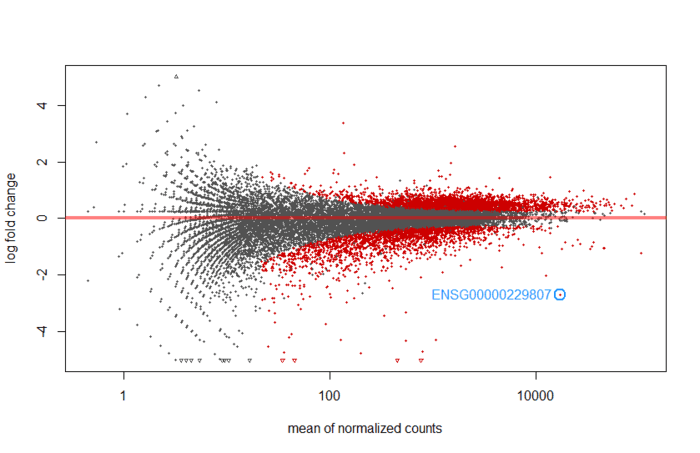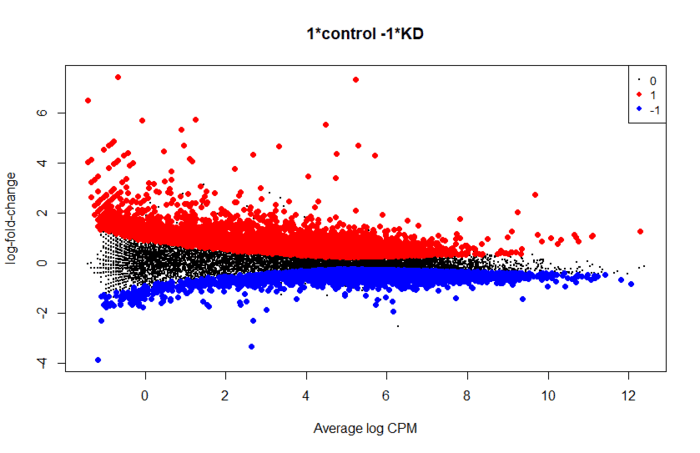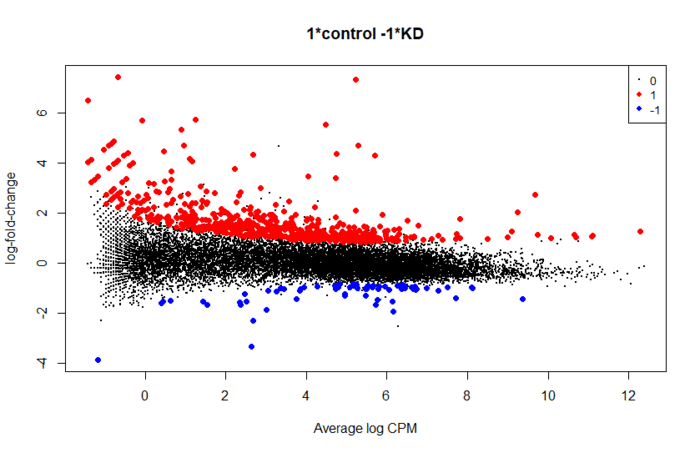# 转录组入门（7）：差异表达分析

1 38,544

## 目录

• 数据填坑
• 理论基础：线性模型， 设计矩阵和比较矩阵
• 标准化一二事
• 探索性分析一二事
• 使用DESeq2进行差异基因分析
• 使用edgeR进行差异基因分析
• 使用limma进行差异基因分析
• 不同软件包分析结果比较
• 使用GFOLD进行无重复样本的差异基因分析
• 不同差异表达分析的比较

## 数据填坑

• 使用edgeR，指定dispersion值
• 无重复转录组数据推荐用同济大学的GFOLD

• 计算KD重复组的均值差，作为泊松分布的均值
• 使用概率函数`rpois()`随机产生一个数值，前一步的均值作为lambda，
```# import data if sample are small
options(stringsAsFactors = FALSE)
sep="\t", col.names = c("gene_id","control"))
sep="\t", col.names = c("gene_id","rep1"))
sep="\t",col.names = c("gene_id","rep2"))
# merge data and delete the unuseful row
raw_count <- merge(merge(control, rep1, by="gene_id"), rep2, by="gene_id")
raw_count_filt <- raw_count[-1:-5,]

ENSEMBL <- gsub("(.*?)\\.\\d*?_\\d", "\\1", raw_count_filt\$gene_id)
row.names(raw_count_filt) <- ENSEMBL
## the sample problem
delta_mean <- abs(mean(raw_count_filt\$rep1) - mean(raw_count_filt\$rep2))

sampleNum <- length(raw_count_filt\$control)
sampleMean <- mean(raw_count_filt\$control)
control2 <- integer(sampleNum)

for (i in 1:sampleNum){
if(raw_count_filt\$control[i] < sampleMean){
control2[i] <- raw_count_filt\$control[i] + abs(raw_count_filt\$rep1[i] - raw_count_filt\$rep2[i])
}
else{
control2[i] <- raw_count_filt\$control[i] + rpois(1,delta_mean)
}
}
raw_count_filt\$control2 <- control2```

## 理论基础：线性模型， 设计矩阵和比较矩阵

A1............
A2............
A3...... .........

：方差分析(Analysis of Variance, ANAOVA)名字听起来好像是检验方差，但其实是为了判断样本之间的差异是否真实存在，为此需要证明不同处理内的方差显著性大于不同处理间的方差。

sample1control
sample2control
sample3KD
sample4KD

## 使用DESeq2进行差异基因分析

DESeq2要求的数据是raw count， 没必要进行FPKM/TPM/RPFKM/TMM标准化。

functionpackageframeworkoutputDESeq2 input function
summarizeOverlapsGenomicAlignmentsR/BioconductorSummarizedExperimentDESeqDataSet
tximporttximportR/Bioconductorlist of matricesDESeqDataSetFromTximport
htseq-countHTSeqPythonfilesDESeqDataSetFromHTSeq

```library(DESeq2)
countData <- raw_count_filt[,2:5]
condition <- factor(c("control","KD","KD","control"))
dds <- DESeqDataSetFromMatrix(countData, DataFrame(condition), design= ~ condition )```

： 这一步到下一步之间可以过滤掉一些low count数据，节省内存，提高运行速度

```nrow(dds)
dds <- dds[ rowSums(counts(dds)) > 1, ]
nrow(dds)```

```dds <- DESeq(dds)
# 出现如下提示信息，说明运行成功
estimating size factors
estimating dispersions
gene-wise dispersion estimates
mean-dispersion relationship
final dispersion estimates
fitting model and testing```

``````res <- results()
``````

```mcols(res, use.names = TRUE)
DataFrame with 6 rows and 2 columns
type                                     description
<character>                                     <character>
baseMean       intermediate       mean of normalized counts for all samples
log2FoldChange      results log2 fold change (MLE): condition KD vs control
lfcSE               results         standard error: condition KD vs control
stat                results         Wald statistic: condition KD vs control
pvalue              results      Wald test p-value: condition KD vs control

```summary(res)
out of 29469 with nonzero total read count
LFC > 0 (up)     : 3154, 11%
LFC < 0 (down)   : 2095, 7.1%
outliers      : 0, 0%
low counts    : 15111, 51%
(mean count < 22)
 see 'cooksCutoff' argument of ?results
 see 'independentFiltering' argument of ?results```

An MA plot is an application of a Bland–Altman plot for visual representation of genomic data. The plot visualises the differences between measurements taken in two samples, by transforming the data onto M (log ratio) and A (mean average) scales, then plotting these values. Though originally applied in the context of two channel DNA microarray gene expression data, MA plots are also used to visualise high-throughput sequencing analysis --From wikipeida
M表示log fold change，衡量基因表达量变化，上调还是下调。A表示每个基因的count的均值。根据summary可知，low count的比率很高，所以大部分基因表达量不高，也就是集中在0的附近（log2(1)=0，也就是变化1倍）.提供了模型预测系数的分布总览。

```plotMA(res, ylim = c(-5,5))
with(res[topGene, ], {
points(baseMean, log2FoldChange, col="dodgerblue", cex=2, lwd=2)
text(baseMean, log2FoldChange, topGene, pos=2, col="dodgerblue")
})``````res.shrink <- lfcShrink(dds, contrast = c("condition","KD","control"), res=res)
plotMA(res.shrink, ylim = c(-5,5))
with(res[topGene, ], {
points(baseMean, log2FoldChange, col="dodgerblue", cex=2, lwd=2)
text(baseMean, log2FoldChange, topGene, pos=2, col="dodgerblue")
})````res.deseq2 <- subset(res, padj < 0.05)`

## 使用edgeR进行差异基因分析

edgeR使用`DGEList`函数读取count matrix数据，也就说你需要提供一个现成的matrix数据，而不是指望它能读取单独的文件，然后进行合并(当然机智的我发现，其实可以用 tximportDESeqDataSetFromHTSeq 读取单独的文件，然后传递给`DGEList`)

```library(edgeR)
group <- factor(c("control","KD","KD","control"))
genelist <- DGEList(counts=raw_count_filt[,2:5], group = group)```

```# 简单粗暴的方法
keep <- rowSums(genelist\$count) > 50
# 利用CPM标准化
keep <- rowSums(cpm(genelist) > 0.5 ) >=2
table(keep)
genelist.filted <- genelist[keep, ,keep.lib.sizes=FALSE]```

`genelist.norm <- calcNormFactors(genelist.filted)`

```design <- model.matrix(~0+group)
colnames(design) <- levels(group)
design
control KD
1       1  0
2       0  1
3       0  1
4       1  0```

```genelist.Disp <- estimateDisp(genelist.norm, design, robust = TRUE)
plotBCV(genelist.Disp)```

```fit <- glmQLFit(genelist.Disp, design, robust=TRUE)

```cntr.vs.KD <- makeContrasts(control-KD, levels=design)
res <- glmQLFTest(fit, contrast=cntr.vs.KD)
ig.edger <- res\$table[p.adjust(res\$table\$PValue, method = "BH") < 0.01, ]```

```topTags(res,n=10)
is.de <- decideTestsDGE(res)
summary(is.de)
plotMD(res, status=is.de, values=c(1,-1), col=c("red","blue"),
legend="topright")``````tr <- glmTreat(fit, contrast=B.LvsP, lfc=log2(1.5))
s```## 使用limma进行差异分析

• 原始数据过滤，根据标准化read count 或者 raw count 作为筛选标准
• 通过各种算法（如经验贝叶斯，EM）预测dispersion离散值
• 广义线性模型拟合数据
• 差异分析，也就是统计检验部分

Limma原先用于处理基因表达芯片数据，可是说是这个领域的老大 :sunglasses: 。如果你仔细看edgeR导入界面，你就会发现，edgeR有一部分功能依赖于limma包。Limma采用经验贝叶斯模型（ Empirical Bayesian model）让结果更稳健。

• 精确权重法（precision weights）也就是“voom"
• 经验贝叶斯先验趋势（empirical Bayes prior trend），也就是”limma-trend“

```library(edgeR)
library(limma)
group <- factor(c("control","KD","KD","control"))
genelist <- DGEList(counts=raw_count_filt[,2:5], group = group)

### filter base  use CPM
keep <- rowSums(cpm(genelist) > 0.5 ) >=2
table(keep)
genelist.filted <- genelist[keep, ,keep.lib.sizes=FALSE]
### normalizaition
x <- calcNormFactors(x, method = "TMM")```

```design <- model.matrix(~0+group)
colnames(design) <- levels(group)
logCPM <- cpm(genelist.norm, log=TRUE, prior.count=3)
fit <- lmFit(logCPM, design)
fit <- eBayes(fit, trend=TRUE)
topTable(fit, coef=ncol(design))```

```### DGE with voom
v <- voom(genelist.norm, design, plot=TRUE)
#v <- voom(counts, design, plot=TRUE)
fit <- lmFit(v, design)
fit <- eBayes(fit)
all <- topTable(fit, coef=ncol(design), number=10000)
sig.limma <- all[all\$adj.P.Val < 0.01, ]
fit <- treat(fit, lfc=log2(1.2))
topTreat(fit, coef=ncol(design))```

### 不同软件包分析结果比较

```library(UpSetR)
input <- fromList(list(edgeR=rownames(sig.edger), DESeq2=rownames(sig.deseq2), limma=rownames(sig.limma)))```

## 参考文件

 Comparing the normalization methods for the differential analysis of Illumina high-throughput RNA-Seq data

• 本文由 发表
• 转载请务必保留本文链接：https://www.plob.org/article/11506.html

• hyp 3

转录组入门（7）：差异表达分析 学习